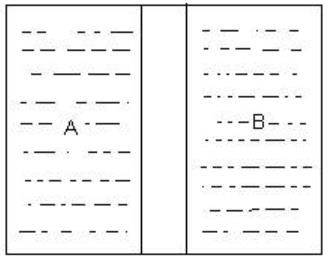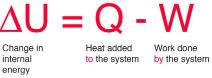Courses

# Test: First Law of Thermodynamics

## 10 Questions MCQ Test Physics For JEE | Test: First Law of Thermodynamics

Description
This mock test of Test: First Law of Thermodynamics for JEE helps you for every JEE entrance exam. This contains 10 Multiple Choice Questions for JEE Test: First Law of Thermodynamics (mcq) to study with solutions a complete question bank. The solved questions answers in this Test: First Law of Thermodynamics quiz give you a good mix of easy questions and tough questions. JEE students definitely take this Test: First Law of Thermodynamics exercise for a better result in the exam. You can find other Test: First Law of Thermodynamics extra questions, long questions & short questions for JEE on EduRev as well by searching above.
QUESTION: 1

### The Zeroth law leads to the concept of

Solution:

Zeroth law states that if two thermodynamic systems are each in thermal equilibrium with a third one, then they are in thermal equilibrium with each other. Thus as it deals with thermal equilibrium it is very clear that it is a concept of temperature.

QUESTION: 2

### Suppose we have a box filled with gas and a piston is also attached at the top of the box.What are the ways of changing the state of gas (and hence its internal energy)?

Solution:

As change of state depends on both temperature and pressure, so, either method A or C can be used. We can understand this by ideal gas equation PV = nRT

QUESTION: 3

### Which of the following statement is true for a thermodynamical system where ∆U is the increase in internal energy and ∆W work done respectively?

Solution:

∆ U = ∆ Q - ∆ W
In an adiabatic process ∆Q is zero, therefore
∆ U = - ∆ W

QUESTION: 4

Hot coffee in a thermos flask is shaken vigorously, considering it as a system which of the statement is not true?

Solution:

No, heat is not transferred as the flask is insulated from the surroundings ∴dQ=0

QUESTION: 5

The internal energy and the work done by a system decreases by same amount then

Solution:

The internal energy of a system decreases by the same amount as the work done by the system.

Change in internal energy=work done+heat exchange
change in internal energy=work done if process has no heat exchange, i.e. it's adiabatic and the temperature must decrease

QUESTION: 6

Which wall would allow the flow of thermal energy between systems A and B to achieve thermal equilibrium?Solution:

Wall that permits *heat" to flow through them,such as engine block is called diathermic wall.
wall Perfectly insulating ball that doesn't allow the flow heat to them are called adiabatic walls.

QUESTION: 7

The first law of thermodynamics

1. Is a restatement of the principle of conservation of energy as applied to heat energy
2. Is the basis for the definition of internal energy
3. Is basis for the definition of temperature
4. asserts the impossibility of achieving an absolute zero temperature.

Solution:

The change is internal energy if the system is equal to the difference between the heat added to the system and work done by the system.QUESTION: 8

The First Law of Thermodynamics states that:

Solution:

The first law of thermodynamics states that the total energy of an isolated system is constant. Energy can be transformed from one form to another, but can neither be created nor destroyed.

According to this law, some of the heat given to system is used to change the internal energy while the rest in doing work by the system. Mathematically,

ΔQ=ΔU+ΔW

where,

ΔQ =  Heat supplied to the system

ΔW= Work done by the system.

ΔU = Change in the internal energy of the system.

If Q is positive, then there is a net heat transfer into the system, if  W is positive, then there is work done by the system. So positive Q adds energy to the system and positive W takes energy from the system.

It can also be represented as  ΔU=ΔQ-ΔW

We can say that internal energy tends to increase when heat is given to the system and vice versa.

QUESTION: 9

Internal energy of a system increases by 60 J when 140 Jof heat is added to the gaseous system. The amount of work done would be:

Solution:

We know that dq = dU + dW
And as dU =  60 and dq = 140J
We get dW = 140 - 60 = 80J

QUESTION: 10

First law of thermodynamics tells us:

Solution:

First law of thermodynamics is based on law of conservation of energy i.e. energy supplied to a system has to be used in raising the internal energy of the system. So heat supplied is used to carry out the process.
∆U = Q + W.